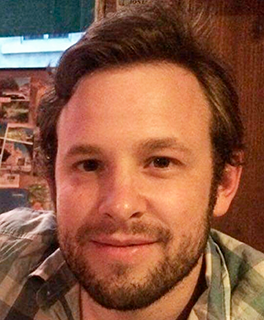# Events

Francisco Vial: “Adaptively Learning the Parallelepiped: A Key-Recovery Attack Against the First Fully Homomorphic Cryptosystem”
08/NovAbstract: In a groundbreaking series of articles, Craig Gentry proposed in 2009 the first fully homomorphic encryption scheme. In the first variation of the scheme, secret keys are bases of polynomial ideal lattices, which provide algebraic structures that can be exploited by an attacker. In this talk, we introduce the Adaptively Learning the Parallelepiped problem (ad-LP), and show its relation to the problem of extracting a secret key of Gentry’s scheme, given bounded access to a decryption oracle. We then describe a geometric algorithm that learns an approximation of the parallelepiped using only O(n log(n)^3) decryptions (where n is the dimension of the lattice), and a practical depth-first search version. We use an implementation to demonstrate the attack using a standard CPU with C++, GMP, and Sage, which extracted secret keys in dimension 334 (safe against lattice reduction techniques) with 87,000 decryption queries in about 15 minutes. We also discuss some countermeasures and extensions of the attack against other cryptographic schemes.

Date: November 8th, 2018. 10.00 h.

Venue: Sala de Consejo, Depto. de Ciencia de la Computación, P. Universidad Católica.